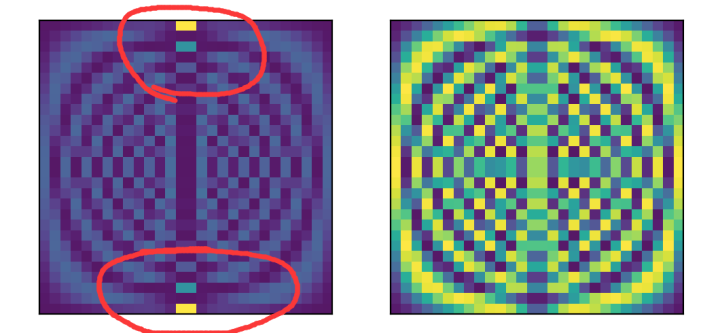CS界最火热的莫过于人工智能技术了，其中最有趣的就是神经病网络；

https://www.nature.com/articles/nphys4037.pdf?origin=ppubwww.nature.com

SSH的哈密顿量很简单，

diag1 = np.array(
[1 + delta_t*(-1)**(i) for i in range(2*L-1)],
dtype = complex,
)
H = np.diag(diag1,-1) +np.diag(diag1,1)

w,v = blas.eigh(H)
N=np.real(np.conj(v)*v)

class AutoEncoder(nn.Module):
def __init__(self):
super(AutoEncoder, self).__init__()

self.encoder = nn.Sequential(
nn.Linear(4*L*L, 128),
nn.ReLU(),
nn.Linear(128, 64),
nn.ReLU(),
nn.Linear(64, 2),
)
self.decoder = nn.Sequential(
nn.Linear(2, 64),
nn.ReLU(),
nn.Linear(64, 128),
nn.ReLU(),
nn.Linear(128, 4*L*L),
)

def forward(self, x):
encoded = self.encoder(x)
decoded = self.decoder(encoded)
return encoded, decoded

1标记的是有拓扑的，0标记的是没有拓扑的地方；收敛的很快，为了大家方便，我特意炼丹到一个比较慢的学习步骤上去，得到：

1、Qi Xiao-Liang 指出，[1712.01850] Determining a local Hamiltonian from a single eigenstate 对于一局域哈密顿的单一的本征态，就几乎足够得到整个哈密顿了。看起来很诡异，但是通过关联谱函数的研究，我们看到，事实确实如此。试构建合适的人工智障，基于单个本征态去判断体系是否有能隙。

2、对于相互作用体系，我们能否如此进行拓扑分类？对于非厄米体系呢？

3、试利用RNN去处理TEBD的前一段数据，证明，RNN对于某些体系确实具有预测能力。

4、试证明，上面的自炼丹过程(auto-encode)确实能够区分MBL态，无论这个态的数据来自ED还是来自DRMG-X。

Email: caidish艾特hust.edu.cn

Blog: https://physcai.com